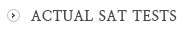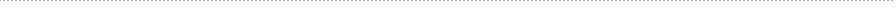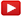칼리지보드 SAT 동영상 강의

### New SAT Math - Heart Of Algebra

• Solving linear equations and linear inequalities
• Linear equation word problems
• Linear inequality word problems
• Systems of linear inequalities word problems
• Solving systems of linear equations
• Systems of linear equations word problems

### New SAT Math - Passport to Advanced Mathematics

• Interpreting nonlinear expressions
• Quadratic and exponential word problems
• Manipulating quadratic and exponential expressions
• Radicals and rational exponents
• Radicals and rational equations
• Operations with rational expressions
• Nonlinear equation graphs

### Problem Solving and Data Analysis

• Ratios, rates, and proportions
• Center, spread, and shape of distribution
• Data collection and conclusions

### New SAT Math - Additional Topics in Math

• Angles, arc lengths, and trig functions